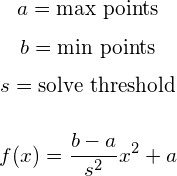# Dynamic Value

A dynamic value challenge is a challenge whose point value decreases after each solve. By reducing the value of the challenge on each solve, all users who have previously solved the challenge will have lowered scores. Thus an easier and more solved challenge will naturally have a lower point value than a harder and less solved challenge.

info

Hidden users and teams will not affect the scoring of dynamic value challenges. If a hidden user or team (e.g. an admin) solves a dynamic value challenge, the value for other participants will remain unchanged.

Within CTFd you are free to mix and match regular and dynamic challenges.

The current implementation requires the challenge to keep track of three values:

• Initial - The original point valuation
• Decay - The amount of solves before the challenge will be at the minimum
• Minimum - The lowest possible point valuation

The value decay logic is implemented with the following math:or in pseudo code:

``value = (((minimum - initial) / (decay ** 2)) * (solve_count ** 2)) + initialvalue = math.ceil(value)``

If the number generated is lower than the minimum, the minimum is chosen instead.

CTFd uses a parabolic function instead of a linear, exponential, or logarithmic decay function so that higher valued challenges have a slower drop from their initial value.

## Value Decay Simulator​

Below is a simulator that allows you to test out the decay function variables of your dynamic value challenge.